Flex SensorWorking Principle:

A flex sensor or bend sensor is a sensor that measures the amount of deflection or bending. This flex sensor is a variable resistor like no other. The resistance of the flex sensor increases as the body of the component bends. Usually, the sensor is stuck to the surface, and resistance of sensor element is varied by bending the surface.

How it Works:

One side of the sensor is printed with a polymer ink that has conductive particles embedded in it. When the sensor is straight, the particles give the ink a resistance of about 30k Ohms. When the sensor is bent away from the ink, the conductive particles move further apart, increasing this resistance (to about 50k-70K Ohms when the sensor is bent to 90°, as in the diagram below).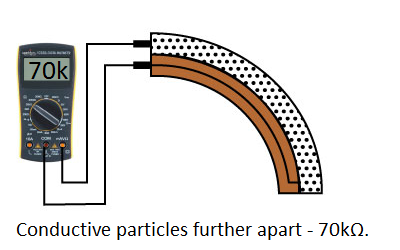When the sensor straightens out again, the resistance returns to the original value. By measuring the resistance, you can determine how much the sensor is being bent.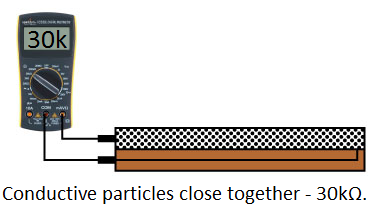Types of flex sensor:

Flex sensors are available in two sizes:

Features:

• Angle Displacement Measurement
• Bends and Flexes physically with motion device
• Simple Construction
• Low Profile

Specifications:

• Life Cycle: >1 million
• Height: 0.43mm (0.017″)
• Temperature Range: -35°C to +80°C
• Flat Resistance: 25K OhmsResistance
• Tolerance: ±30%
• Bend Resistance Range: 45K to 125K Ohms
• Power Rating : 0.50 Watts continuous. 1 Watt Peak

https://www.sparkfun.com/datasheets/Sensors/Flex/flex22.pdf

Do’s and Don’ts :

The flex sensor is designed to be flexed in just one direction – away from the ink – as demonstrated in the image below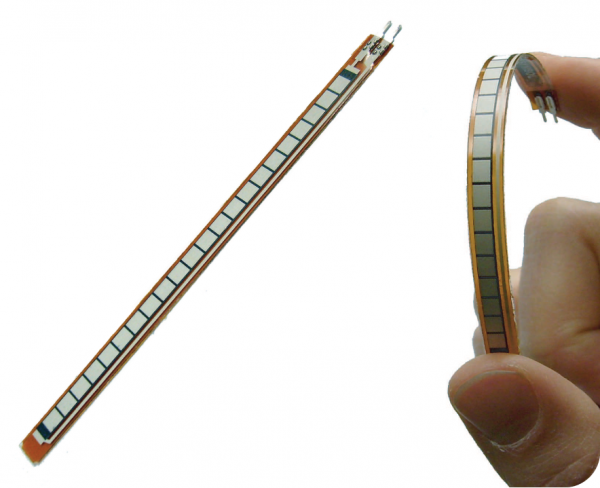Bending the sensor in the other direction will not produce any reliable data, and may damage the sensor. Also take care not to bend the sensor close to the base, as they have a tendency to kink and fail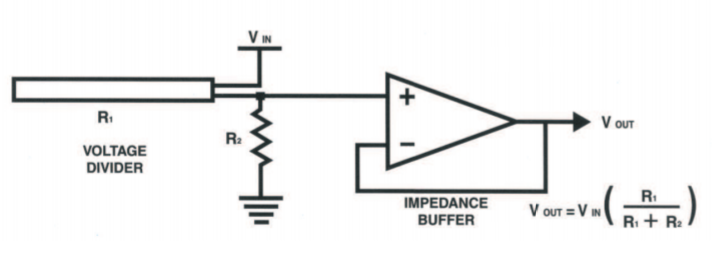Connections with Arduino: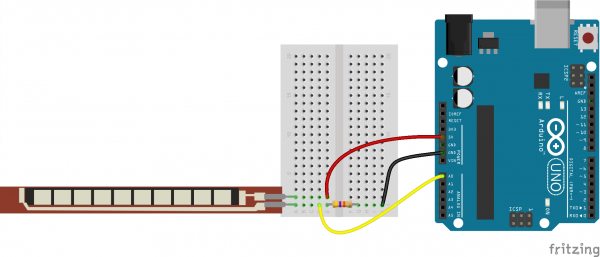Arduino Code:

const int FLEX_PIN = A0; // Pin connected to voltage divider output

const float VCC = 4.98; // Measured voltage of Ardunio 5V line

const float R_DIV = 47500.0; // Measured resistance of 3.3k resistor

// Upload the code, then try to adjust these values to more

// accurately calculate bend degree.

const float STRAIGHT_RESISTANCE = 37300.0; // resistance when straight

const float BEND_RESISTANCE = 90000.0; // resistance at 90 deg

void setup()

{

Serial.begin(9600);

pinMode(FLEX_PIN, INPUT);

}

void loop(){

float flexV = flexADC * VCC / 1023.0;

float flexR = R_DIV * (VCC / flexV – 1.0);

Serial.println(“Resistance: ” + String(flexR) + ” ohms”);

// Use the calculated resistance to estimate the sensor’s

// bend angle:

float angle = map(flexR, STRAIGHT_RESISTANCE, BEND_RESISTANCE,

0, 90.0);

Serial.println(“Bend: ” + String(angle) + ” degrees”);

Serial.println();

delay(500);}

Reference:

https://learn.sparkfun.com/tutorials/flex-sensor-hookup-guide

http://ethesis.nitrkl.ac.in/7202/1/To_Suna_2015.pdf

http://www.instructables.com/id/How-to-use-a-Flex-Sensor-Arduino-Tutorial/

https://www.sparkfun.com/datasheets/Sensors/Flex/flex22.pdf